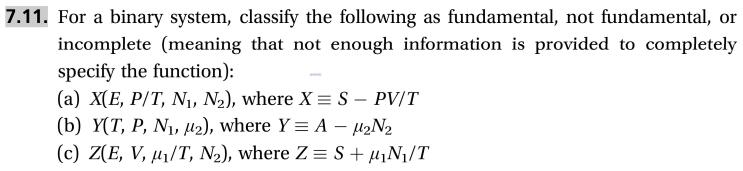# 7.11. For a binary system, classify the followingincomplete (meaning that not enough information is provided to completelyas fundamental, not fundamental,orspecify the function):(a) X(E, P/T, Ni, N2), where X S - PV/T(b) Y(T, P, N, 2), where Y A H2N2(c) Z(E, V, u/T, N2), where Z S+N/T

Question
68 views

This problem is (7.11) from a book  "Thermodynamics and Statistical Mechanics An Integrated Approach by M. Scott Shell"help_outlineImage Transcriptionclose7.11. For a binary system, classify the following incomplete (meaning that not enough information is provided to completely as fundamental, not fundamental, or specify the function): (a) X(E, P/T, Ni, N2), where X S - PV/T (b) Y(T, P, N, 2), where Y A H2N2 (c) Z(E, V, u/T, N2), where Z S+N/T fullscreen
check_circle

Step 1

(a) The given function is found to be fundamental as S is function of E,V,N which satisfy the given expression.

Step 2

(b) The given function is found to be non-fundamental  as A is f...

### Want to see the full answer?

See Solution

#### Want to see this answer and more?

Solutions are written by subject experts who are available 24/7. Questions are typically answered within 1 hour.*

See Solution
*Response times may vary by subject and question.
Tagged in

### Chemical Engineering